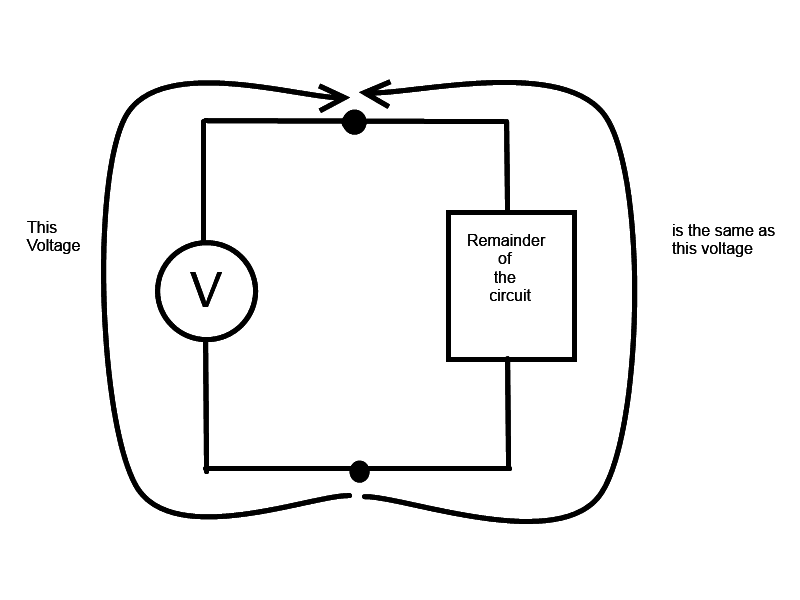# Electromotive Force

The potential difference between the ends of a wire is given by Vab = IR. Combing that with E = Vab we have
E = Vab = IR (ideal source of emf).

" That is, when a positive charge flows around the circuit, the potential rise as it passes through the ideal source is numerically equal to the potential drop as it passes through the remainder of the circuit. Once and are known, this relationship determines the current in the circuit."- I cant seem to understand what this means???

NascentOxygen
Staff Emeritus
Imagine going around a closed loop in the circuit. As charge goes through the resistor it drops down in potential. As it goes through the battery it rises in potential. Those two potentials are equal in magnitude.

•AGGENGR
CWatters
Homework Helper
Gold Member
...the potential rise as it passes through the ideal source is numerically equal to the potential drop as it passes through the remainder of the circuit.

If you think about it this is because the "ideal source" and the "remainder of the circuit" are connect to the same two nodes....•